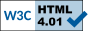## On-line solar and stellar wind graphic calculator

The graphic calculator plots the stationary transonic wind solution of the hydrodynamic equations in spherical symmetry for the case of an ideal gas in a central gravitational potential. The temperature profile is assumed isothermal, i.e. constant and therefore independent of the distance to the center of symmetry. Originally published by E.N. Parker in 1958 (see Parker, 1958) the transonic solution of the fluid equations has been later confirmed to provide a fair approximation of the real solar wind by the numerous spacecraft launched into interplanetary space since the early sixties. Beside the Sun, other stars have hot coronas from where a transonic wind, similar to the solar wind, may emanate.

The calculator provides the ability to plot the radial velocity, the density, the pressure and the electrostatic potential profiles of the wind from a generic star given its mass, coronal temperature and coronal radius (i.e. the distance between the position of the temperature maximum in the corona and the star's center). The total mass flux (or stellar mass loss) is explicitly computed. The wind is assumed to be an ideal gas of singly ionized ions and electrons. The average atomic mass (half of the ion mass plus the electron mass) can be specified by the user. More sophisticated simulations of the solar wind, starting from below the solar corona can be found here.

NB 1: Server may require several seconds to respond to a "submit" action.
NB 2: Connection will fail if you are behind a firewall not allowing access through port 7779 (e.g. on some Wireless Networks).Select action Plot data Get dataChoose parameters (defaults are for typical solar conditions)

 [Units] [Units]Coronal radius [R_Sun] Outer limit [R_cor] Scale LogLin Stellar mass [M_Sun] Grid Atomic mass [m_p] Temperature [106 K] Coronal density [108 cm⁻³]

Reminder: Sun radius: R_Sun= 6.96e8 [m], Solar mass: M_Sun=1.99e30 [kg], Proton mass: m_p=1.67e-27 [kg], Gravitational constant: G=6.673e-11 [m³ kg⁻¹ s⁻²], Boltzmann constant: k=1.38e-23 [m² kg s⁻² K⁻¹]
Filippo Pantellini, Observatoire de Paris, LESIA, 92195 Meudon
Roland Grappin, Ecole Polytechnique, LPP, 91128 Palaiseau(Updated: August 24 2017 19:44 CET) © Observatoire de Paris & Laboratoire de Physique des Plasmas